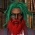Display replies flat, with oldest first Display replies flat, with newest first Display replies in threaded form Display replies in nested formA.S. Losev, "Introduction to worlds of mathematical physics"

## Zoom course for physicists and mathematicians. By Andrey Losev, HSE(Moscow, Russia) and USTC(Hefei, China)

The site of the course is here. Please register here to get zoom link and updates. Course starts on Wednesday, 19 of October, the next class would be 20 of October, and we will have classes all year on Wednesdays and Thursdays. Class would start 11am Moscow that is 4pm Beijing.
Mathematical physics is a science that lies between mathematics and physics. It’s subject are worlds that can be described mathematically and it makes it mathematics. The laws in these worlds are inspired by physics. The method of mathematical physics is to study these worlds altogether like geometry studies all spaces. On the other hand, mathematical physics is believed to be a laboratory from which we can eventually get a theory describing our real world. It is interesting that working in this laboratory people managed to get a lot of purely mathematical conjectures that were later proved to be theorems.

The course would be self contained, I will explained all relevant physics and mathematics. In physics I would start with the Newton’s world, explain it’s incompleteness and restrictions, and we go to fields and least action principle. In particular, we will see that ether theory can be constructed for electrodynamics in dimension 2+1, but not in 3+1. From Einstein-Cartan theory you will understand what spins in electron. We will discuss quantum theory, and the fight of Feynman against Dirac revolution, and the modern understanding of Quantum Field Theory as a monoidal functor between category of cobordisms (equipped with geometrical structure) to category of vector spaces.

Again, the course would be self contained, and I will explain all relevant mathematics including categories and functors, algebrogeometric correspondence, Q-supermanifolds, homological algebra, infinity-algebras and homotopical transfer. So you could appreciate that scattering amplitudes of high energy physics are actually results of the homotopical transfer.